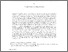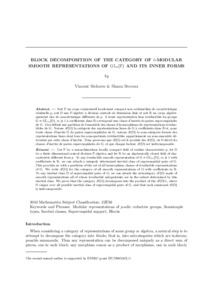# Block decomposition of the category of l-modular smooth representations of GL(n,F) and its inner forms

Sécherre, Vincent and Stevens, Shaun (2016) Block decomposition of the category of l-modular smooth representations of GL(n,F) and its inner forms. Annales Scientifiques de l’École Normale Supérieure, 49 (3). pp. 669-709. ISSN 0012-9593Preview PDF (blocks_final) - Accepted Version Download (421kB) | Preview

## Abstract

Let F be a non-Archimedean locally compact field of residue characteristic p, let D be a finite dimensional central division F-algebra and let R be an algebraically closed field of characteristic different from p. To any irreducible smooth representation of G=GL(m,D) with coefficients in R, we can attach a uniquely determined inertial class of supercuspidal pairs of G. This provides us with a partition of the set of all isomorphism classes of irreducible representations of G. We write R(G) for the category of all smooth representations of G with coefficients in R. To any inertial class O of supercuspidal pairs of G, we can attach the subcategory R(O) made of smooth representations all of whose irreducible subquotients are in the subset determined by this inertial class. We prove that R(G) decomposes into the product of the R(O), where O ranges over all possible inertial class of supercuspidal pairs of G, and that each summand R(O) is indecomposable.

Item Type: Article modular representations of p-adic reductive groups,semisimple types,inertial classes,supercuspidal support,blocks Faculty of Science > School of Mathematics Pure Connector 23 Sep 2016 23:35 24 Oct 2022 06:32 https://ueaeprints.uea.ac.uk/id/eprint/59725 10.24033/asens.2293View Item